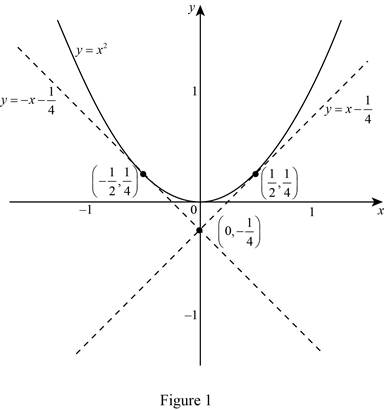# To draw: A diagram showing two perpendicular tangent lines to the parabola that intersect on the y -axis.### Single Variable Calculus: Concepts...

4th Edition
James Stewart
Publisher: Cengage Learning
ISBN: 9781337687805### Single Variable Calculus: Concepts...

4th Edition
James Stewart
Publisher: Cengage Learning
ISBN: 9781337687805

#### Solutions

Chapter 3.1, Problem 72E
To determine

## To draw: A diagram showing two perpendicular tangent lines to the parabola that intersect on the y-axis.

Expert Solution

The two perpendicular tangent lines to the parabola that intersect on the y-axis at the point (0,14)

### Explanation of Solution

Given:

The parabola is y=x2.

Graph:

First, draw the graph of the given parabolic curve y=x2.

Then, draw two perpendicular tangent lines to the parabola that intersects on the y-axis as shown in Figure 1.From Figure 1, it is observed that the two lines y=x14and y=x14 are the tangent to the curve y=x2 intersect on y-axis at the point (0,14).

### Have a homework question?

Subscribe to bartleby learn! Ask subject matter experts 30 homework questions each month. Plus, you’ll have access to millions of step-by-step textbook answers!